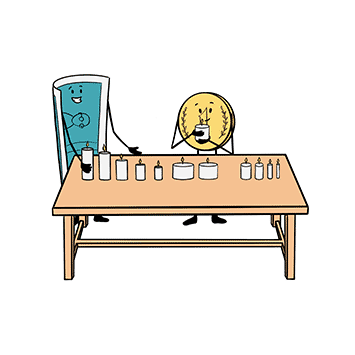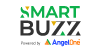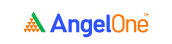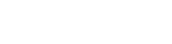# How to Analyze a Profit and Loss Statements

4.8In the previous lesson, we looked at a profit and loss statement and went through the entire process of reading it step-by-step. Now that you’re aware of the various terms used in a P&L statement and their meanings, it is time for us to move on to analysing the statement.

In this segment, we’ll be delving deeper into the P&L statement and use financial ratios to analyse and interpret the financial data of a company. But before we jump in, there’s one key thing that you need to understand. Financial ratios on their own may not make much sense. You’ll have to compare these ratios with those of the previous years or with that of a similar company to fully comprehend the growth of the company you’re evaluating.

For the sake of continuity, we’ll be taking up the profit and loss statement of Hindustan Unilever Limited (HUL) for the financial year 2019-2020 for all our ratio calculations. For your reference, here’s a snapshot of the company’s P&L.## Important financial numbers, ratios, and their calculations

Since there are so many financial ratios, experts have classified them into four broad categories:

• Profitability ratios
• Leverage ratios
• Valuation ratios
• Operating ratios.

They use numbers from both the P&L statement and the balance sheet. So, for better reading clarity, we’ll first take up only the ratios and numbers that you can calculate using the information from the P&L statement.

## 1. Operating revenue

The earnings that are derived from a company’s primary operations are classified as operating revenue. It tells you just how much a company earns through its business. It is calculated using the following formula.

 Operating revenue = total income - other income

In the case of HUL, the operating revenue is clearly indicated in its P&L statement itself: Rs. 38,785 crores.## 2. Operating expenses

Operating expenses show the costs a company has incurred for its primary course of business. It can be easily calculated using this formula.

 Operating expenses = total expenses - finance costs - depreciation and amortisation

Let’s apply this formula to Hindustan Unilever’s profit and loss statement to know just how much operating expenses it incurred during the current year and the previous year.

Operating expenses of HUL (for the year 2019-2020) = Rs. 29,185 crores

(Rs. 30,229 crores - Rs. 106 crores - Rs. 938 crores)Operating expenses of HUL (for the year 2018-2019) = Rs. 29,587 crores

(Rs. 30,139 crores - Rs. 28 crores - Rs. 524 crores)

## 3. Earnings before interest, tax, depreciation, and amortisation (EBITDA)

EBITDA is a very important metric that is used to determine a company’s overall financial performance. Unlike the PBT or PAT, which only tells you the profits made by a company, EBITDA gives you a fair idea of the company’s ability to generate revenue.

Going by this, the higher the EBITDA, the better the company’s financial performance generally is. It is calculated using the following formula.

 EBITDA = profit after taxes (PAT) + taxes + finance costs + depreciation and amortisation

There’s also another formula that you can use to compute EBITDA. It goes like this:

 EBITDA = total operating revenue - total operating expenses

Let’s apply the second formula to HUL’s profit and loss statement to find out the company’s EBITDA for the current year (2019-2020).

EBITDA of HUL (for the year 2019-2020) = Rs. 9,600 crores

(Rs. 38,785 crores - Rs. 29,185 crores)

This standalone figure of EBITDA for HUL doesn’t mean much. In order to make sense of how much the company’s earnings have grown, let’s compare this figure with that of the previous year.

EBITDA of HUL (for the year 2018-2019) = Rs. 8,637 crores

(Rs. 38,224 crores - Rs. 29,587 crores)

Comparing these two figures, we can clearly see that HUL’s EBITDA has grown by Rs. 963 crores (Rs. 9,600 crores - Rs. 8,637 crores), which is a good sign.

## 4. EBITDA margin

The EBITDA margin is a useful financial ratio that helps you assess a company’s efficiency. This ratio shows just how much of a company’s profit is derived from its primary operations. It is expressed as a percentage. A high EBITDA margin denotes that a greater portion of a company’s profits come from its operations. This essentially means that the company is considered to be efficient.

It can be calculated using this formula.

 EBITDA margin in % = (EBITDA ÷ total sales) x 100

By applying this formula, we’ll try to find out Hindustan Unilever’s EBITDA margin for the current year. Since we already know the values of both EBITDA and total sales of HUL, we’ll merely substitute them in the formula.

EBITDA margin (for the year 2019-2020) in % = 25.08%

[(Rs. 9,600 crores ÷ Rs. 38,273 crores) x 100]Let’s now compute the EBITDA margin for the previous year.

EBITDA margin (for the year 2018-2019) in % = 22.93%

[(Rs. 8,637 crores ÷ Rs. 37,660 crores) x 100]Upon comparing these two values, the EBITDA margin of HUL has actually increased by 2.15%, indicating that the company has become more efficient.

## 5. Profit after taxes (PAT) margin

The PAT margin is expressed as a percentage, and it’s one of the simplest financial ratios to compute. It is also among the most useful, since it helps you determine the overall profitability of a company. The greater the PAT margin, the better the profitability of a company.

It can be easily calculated using this formula.

 PAT margin in % = (PAT ÷ total sales) x 100

In the case of Hindustan Unilever, by simply substituting these two values, we can come up with the PAT margin of HUL for the current year.

PAT margin (for the year 2019-2020) in % = 17.60%

[(Rs. 6,738 crores ÷ Rs. 38,273 crores) x 100]Let’s see by how much the PAT margin of HUL has grown in one year.

PAT margin (for the year 2018-2019) in % = 16.02%

[(Rs. 6,036 crores ÷ Rs. 37,660 crores) x 100]The percentage of profitability of Hindustan Unilever has increased by around 1.58% in one year, which is rather commendable.

## 6. Interest coverage ratio

Also known as debt service ratio, this ratio allows you to figure out just how easily a company can pay its debt interest payments. The ratio essentially shows you how much revenue is generated for every rupee of debt interest payment made by a company. The larger the interest coverage ratio, the more capable a company is when it comes to paying off its debt. The formula used to calculate the ratio is as follows.

 Interest coverage ratio = EBIT ÷ finance costs

Where,

EBIT (earnings before interest and taxes) = EBITDA - depreciation and amortisation

Let’s try and find out the interest coverage ratio of Hindustan Unilever for the current year.

Since we already know the EBITDA and depreciation and amortisation expenses, we can simply substitute these values and arrive at EBIT.

EBIT of HUL (for the year 2019-2020) = Rs. 8,662 crores

(Rs. 9,600 crores - Rs. 938 crores)

Interest coverage ratio of HUL (for the year 2019-2020) = 81.72

(Rs. 8,662 crores ÷ Rs. 106 crores)An interest coverage ratio of 81.72 means that the company generates 81.72 times revenue for every single rupee of debt interest payment.

## 7. Cost of goods sold (COGS)

Cost of goods sold is the cost incurred by a company for purchasing or manufacturing its finished goods. COGS includes all the costs incurred towards the production and acquisition of the said finished goods including raw materials, labour, and overheads. The formula to calculate COGS is as follows.

 COGS = cost of materials consumed + purchase of stock-in-trade + other expenses in connection with the purchase or production of finished goods (such as power, fuel, light and water)

With respect to Hindustan Unilever, the cost of goods sold for the current year is as follows.

COGS of HUL (for the year 2019-2020) = Rs. 18,177 crores

(Rs. 11,572 crores + Rs. 6,342 crores + Rs. 263 crores)## 8. Operating ratio

The operating ratio is used to determine a company’s operational efficiency. It establishes a relationship between operating expenses and sales. This ratio is typically used to find out just how efficient a company is when it comes to keeping its operating costs low. The lower the operating ratio, the more efficient a company is at keeping the costs low. It is expressed as a percentage of revenue and can be calculated using this formula.

 Operating ratio in % = [(cost of goods sold (COGS) + other operating expenses) ÷ net sales] x 100

Let’s apply this to Hindustan Unilever to determine just how efficient the company is.

In the case of HUL, here are the values for the metrics used in the formula.

• Cost of goods sold (COGS) = Rs. 18,177 crores

• Other operating expenses = Rs. 11,008 crores

(Rs. 1,691 crores - Rs. 121 crores + Rs. 9,701 crores - Rs. 263 crores)

This is calculated as follows:

Employee benefit expenses + changes in inventories of finished goods (including stock-in-trade) and work-in-progress + other expenses (excluding power/fuel/light/water, since that’s already in the COGS value)

• Net sales = Rs. 38,273 crores

For the value of net sales, take a look at note 24 of HUL’s financial statements.So, the operating ratio of HUL (for the year 2019-2020) in % = 76.25%

[(Rs. 18,177 crores + Rs. 11,008 crores) ÷ Rs. 38,273 crores] x 100

To give you some perspective about the implication of this ratio, generally, for a manufacturing concern, an operating ratio of 70% to 80% is considered to be ideal.

### Wrapping up

While these are the numbers and ratios that you can compute using just the information in the P&L statement, there are several other ratios that you can calculate using the data in the P&L statement combined with the information in the balance sheet. And that’s where we’ll be heading to next. It’s time to get to know the next major financial statement - the balance sheet.

### A quick recap

• The earnings that are derived from a company’s primary operations are classified as operating revenue.
• Operating revenue = total income - other income
• Operating expenses show the costs a company has incurred for its primary course of business.
• Operating expenses = total expenses - finance costs - depreciation and amortisation
• EBITDA = profit after taxes (PAT) + taxes + finance costs + depreciation and amortisation
• EBITDA = total operating revenue - total operating expenses
• The EBITDA margin is a useful financial ratio that helps you assess a company’s efficiency. This ratio shows just how much of a company’s profit is derived from its primary operations. It is expressed as a percentage.
• EBITDA margin in % = (EBITDA ÷ total sales) x 100
• The PAT margin is expressed as a percentage, and it’s one of the simplest financial ratios to compute. It is also among the most useful, since it helps you determine the overall profitability of a company.
• PAT margin in % = (PAT ÷ total sales) x 100
• The interest coverage ratio, also known as debt service ratio, allows you to figure out just how easily a company can pay its debt interest payments. The ratio essentially shows you how much revenue is generated for every rupee of debt interest payment made by a company.
• Interest coverage ratio = EBIT ÷ finance costs
• Cost of goods sold (COGS) is the cost incurred by a company for purchasing or manufacturing its finished goods.
• COGS = cost of materials consumed + purchase of stock-in-trade + other expenses in connection with the purchase or production of finished goods (such as power, fuel, light and water)
• The operating ratio is used to determine a company’s operational efficiency. It establishes a relationship between operating expenses and sales.
• Operating ratio in % = [(cost of goods sold (COGS) + other operating expenses) ÷ net sales] x 100Take the quiz for this chapter & mark it complete.

How would you rate this chapter?

Aakanksha Mathur

11 Jun 2021, 10:46 AM

nice content.

Replies (1)

Get Information Mindfulness!

Catch-up With Market

News in 60 Seconds.The perfect starter to begin and stay tuned with your learning journey anytime and anywhere.